## Atom calculations

The software contains pre-calculated atomic presets for ELWF(a-files), so that the changes in the following parameters will not be required. The meanings of the atomic calculation parameters are the following:

•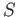- number of not empty electron terms (1s, 2s, 2p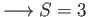). The order of terms is showed on Table 1.
•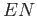- number of points in energy interval, should be set to 400.
•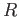- max radius in real space in Ry were excited WF calculated, usually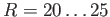.
•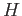- step in logarithmic energy scale, calculated automatically.
•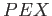- type of exchange-correlation function, 1 stands for Hartree-Fock exact exchange.
•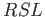- radius of Muffin-Tin sphere used in all further calculations.
•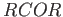- radius of stored core electron states.
•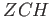- number of not filled electron terms/shells.
•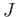- index of changing term,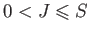.
•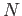,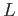,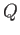- principal, orbital and occupancy of the electron term. For example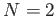,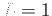,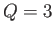means configuration 2p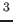. Full explanation are given in Table 1.
•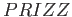- keyword used for treatment of negatively charged atoms, should be 1 one for neutral and positive charged systems.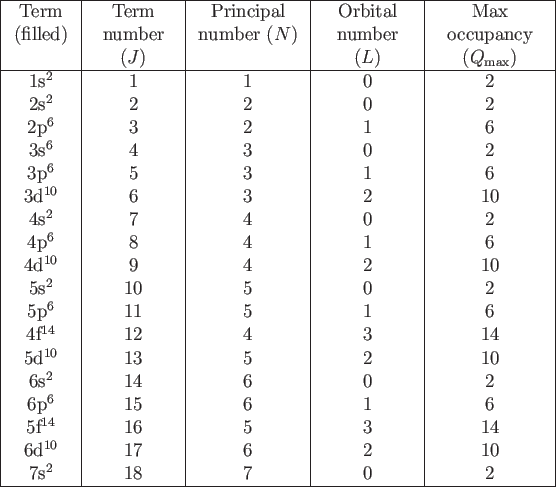By default all terms up to-th are filled with electrons. In order to simulate particular atom one should change several (usually one) last terms by setting appropriate set of parameters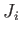,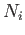,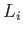,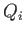,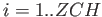.

For example for simulation of Oxygen atom in ground state one should change the occupancy of 2p term from default 2p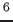to actual 2p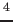by setting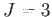,,,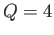in the atom wave function form shown on Figure 4.

In order to get atom with core hole which should correspond to the absorbing atom one should change 1s (K-edge) or 2p (L2,3-edge) term in the same way.

These are a bit annoying and error-rich parts of the calculation and we are working to make presets for all stable atoms to simplify user experience.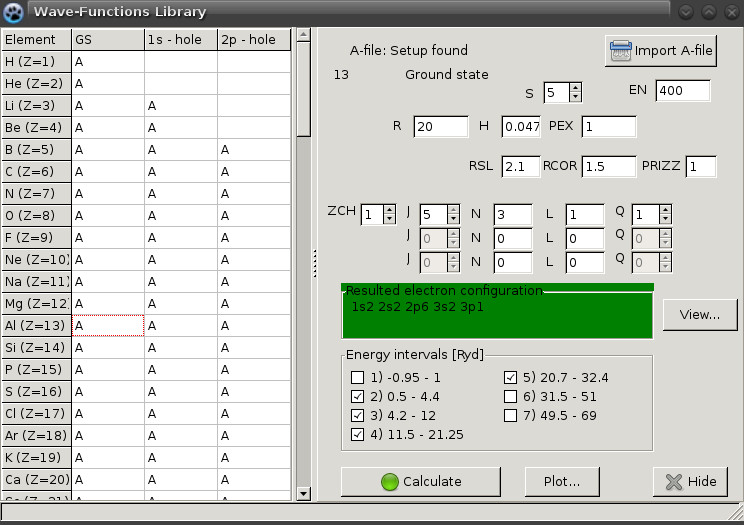The resulting electron configuration could be verified by clicking View button. Window showed on Figure 5 should appear. The first data block here demonstrates accounted electron orbitals and their occupancy while the second shows energy levels of these orbitals (Ry).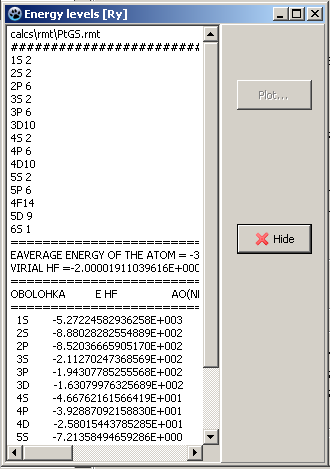The next important quantity is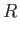SL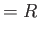MT radii. It should be chosen at the value of total potential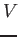C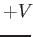XC = -1.1 Ry. According the Figure 6,SL=2.2 Bohr for ground state calculation of Platinum atom.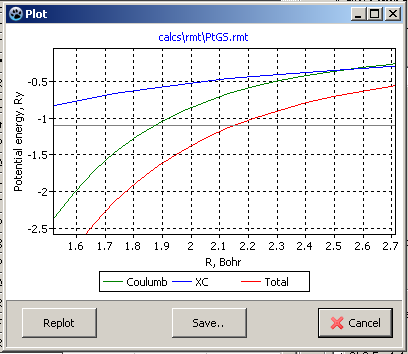After these preparations all WFs may be computed. Just mark boxes corresponding to the calculated energy intervals and press Calculate button. Here ELWF code will be executed several times and B-files should appear in calc/b directory.

These calculation should be completed both for absorbing X-ray atom and his neighbors.

leon 2015-12-15